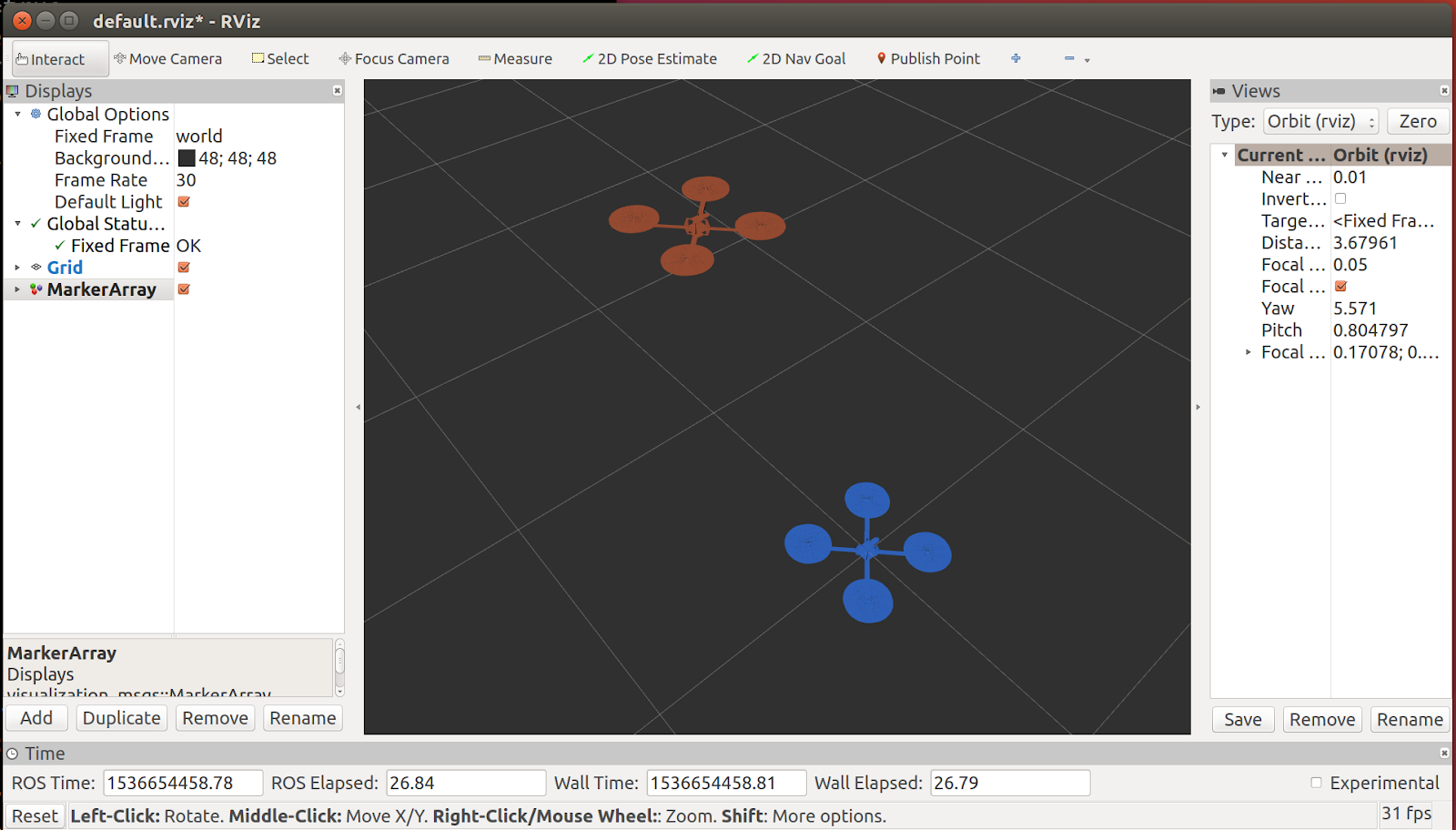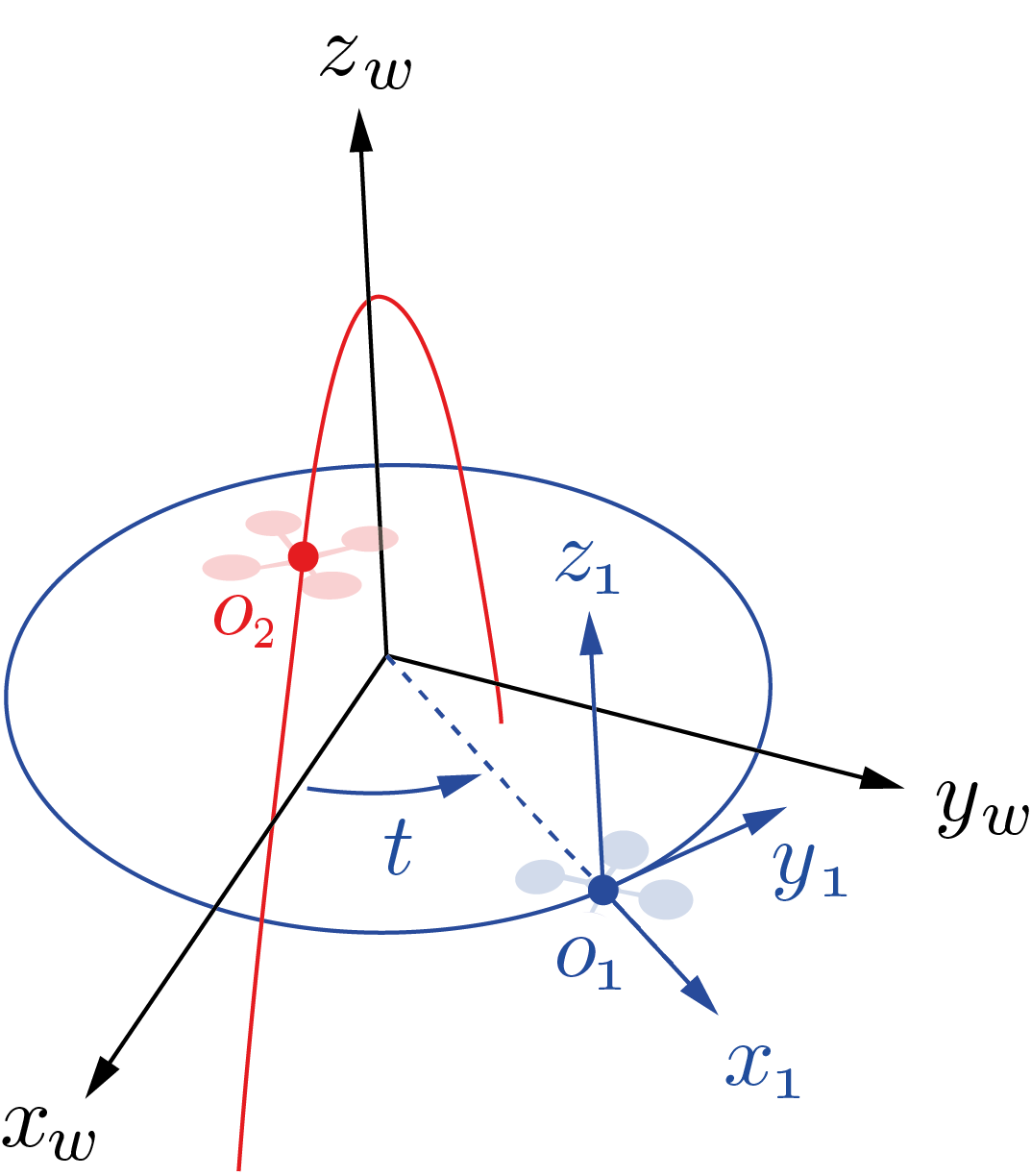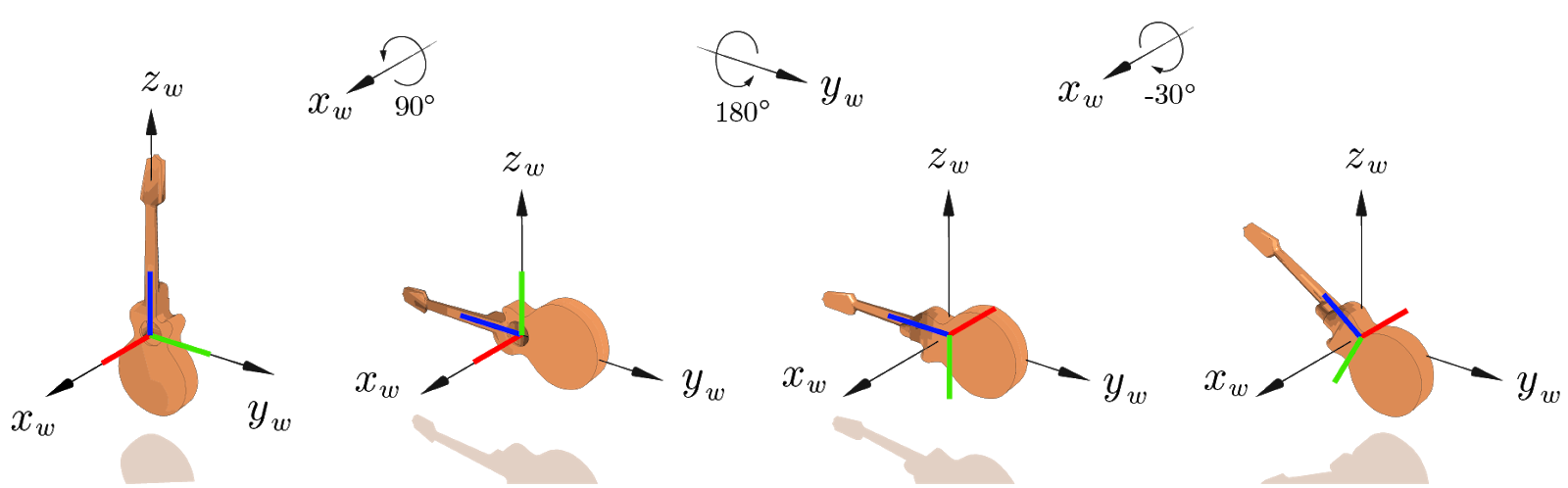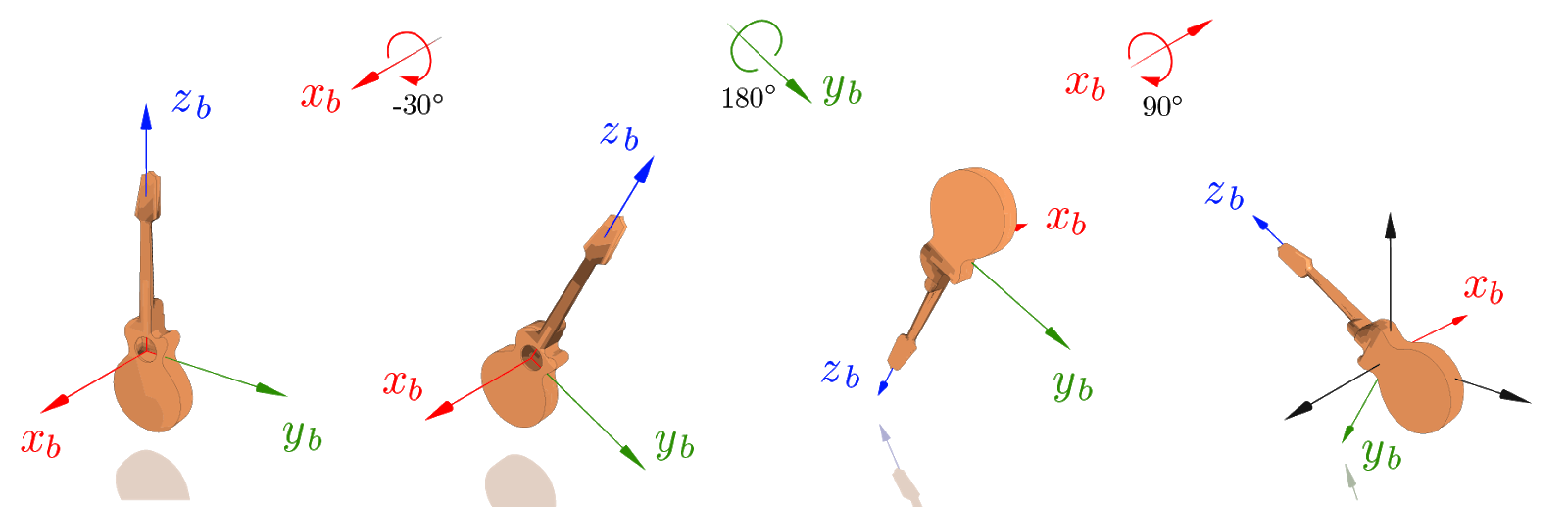# Exercises

## Submission

To submit your solutions create a folder called lab2 and push one or more file to your repository with your answers (it can be plain text, markdown, pdf or whatever other format is reasonably easy to read)

• for math-related deliverables, typeset your responses using LaTeX (or via Word, etc.) and add the relevant files to your respository
• for code-based deliverables, push the source code of the entire package

Deadline: the VNAV staff will clone your repository on Wednesday, September 20th at midnight EDT.

### Find a team

Next lab will have a team section, you should start thinking about your team!

## Find a team.

Please fill it before Wednesday, September 20th.

## Setup workspace

### Create the catkin workspace

To get started, we must create a ROS workspace for the VNAV class. Choose your favorite working directory, we will assume you are creating one in your home directory (i.e ~/). In a terminal, run:

$mkdir -p ~/vnav_ws/src$ cd ~/vnav_ws/

### A two-drone scenario

In this part, we are going to work with 3D Rigid Transformations and with tf2, a basic tool provided by ROS to keep track of multiple coordinate frames over time.

#### The static scenario and rViz

To get started, let’s go back to the VNAV workspace and bring up the two-drones static scenario. In this environment, we have two aerial vehicles, AV1 [blue] and AV2 [red] that are not moving, but it serves as a good starting point! With the VNAV workspace sourced in a terminal, run:

roslaunch two_drones_pkg two_drones.launch static:=True


You should see the following window, which shows the initial positions of the two AVs.This window is rViz, the mighty ROS visualizer! Just like most processes in the ROS ecosystem, rViz is a ROS node. Like all other nodes, rViz can subscribe to ROS topics. However, its specialty is converting them into graphics on your screen to display the state of your robotic system.

As a first experience with rViz, let us:

• Add the visualization of /tf. In the Displays panel, click Add, select the By display type Tab in the pop-up and finally select “TF” and confirm with Ok. You should see all the reference frames, with names and their axes represented in red (x), green (y) and blue (z).
• Save the configuration file. So that we don’t have to repeat the step above every time we launch it! Hit CTRL + s or select File > Save Config.

Other published topics can be added to the visualizer in a similar way.

## Problem formulation

We consider the scenario illustrated in the picture below, where two aerial vehicles, AV1 [blue] and AV2 [red] are following different trajectories: a circle and an arc of parabola, respectively. In the scene, we have highlighted the following elements:

• The world frame $(x_w, y_w, z_w)$
• The AV1 body frame, centered in $O_1$ with axes $(x_1,y_1,z_1)$
• The origin of AV2, denoted with $O_2$#### Positions

In the world frame, AV1 and AV2’s origins are given by:

• $o_1^w = [\text{cos}(t), \text{sin}(t), 0]^T$, and
• $o_2^w = [\text{sin}(t), 0, \text{cos}(2t)]^T$,

where $t$ denotes time.

#### Orientations

We will make the following simplifying assumptions:

• AV1’s reference frame is such that $y_1$ stays tangent to AV1’s trajectory for all $t$ and $z_1$ is parallel to $z_w$ for all $t$ (i.e., equivalently, roll = pitch = 0, yaw = $t$)
• AV2’s reference frame moves with pure translation and we can assume that its axes are parallel to the world axes for all times $t$

Notes

• Given the dynamics of a quadrotor, these motions are dynamically infeasible. However, for the purpose of this lab, we disregard this fact and focus on the study of rigid transformations
• To make the math of this problem more interesting, we chose the $y_1$ axis to point in the direction of motion of the drone. However, do not forget that the standard convention is that $x_1$ should point forward!

In the sequel, we reproduce the above scenario in ROS and study the trajectory of AV2 relative to AV1’s coordinate frame.

## Basic ROS commands

### 📨 Deliverable 1 - Nodes, topics, launch files (10 pts)

With the roslaunch command above we have spawned a number of ROS nodes at the same time. Using your knowledge of ROS, answer the following questions:

1. List the nodes running in the two-drone static scenario.
• Hint: you can directly inspect the launch file or get some help from rqt_graph. You will notice that rViz is initially not shown in it but you can uncheck the Debug option for a full picture, feel free to ignore all /rosout nodes and topics and /rqt_gui_py_* that may appear
2. How could you run the two-drone static scenario without using the roslaunch command? List the commands that you would have to execute, in separate terminals, to achieve the same result.
• Hint: rosrun [...], try things out before finalizing your answer!
3. List the topics that each node publishes / subscribes to. What nodes are responsible for publishing the av1, av2, frames? Which topic causes rViz to plot the drone meshes?
• Hint: uncheck items on the left pane in rViz until the meshes disappear, then check what node is publishing the corresponding topic
4. What changes if we omit static:=True? Why?
• Hint: check out and briefly explain the if and unless keywords in the launch file

## Let’s make things move! Publishing the transforms using tf

After exploring the static scenario, it’s time to implement the motions described in the problem formulation section and visualize them in rViz. With the editor of your choice, open frames_publisher_node.cpp in the src folder of two_drones_pkg. In this file, we provide a basic structure of a ROS node.

### Some Context

Please take your time to familiarize with this code before modifying it. Although not mandatory, the pattern found in it is a very common way to implement ROS nodes:

• The node’s source code is encapsulated into a class, FramesPublisherNode (line 6), which keeps a NodeHandle as a private member.
• In the constructor of the class (lines 14 to 19), one performs operations that need to be executed only once upon startup (e.g. typically, initializing subscribers and publishers),
• Using a Timer (lines 10 and 17), the onPublish() method is called periodically - at a 50Hz - and all the operations within it are performed ad libitum,
• In the body of main() (towards the end of the file):
• the node is registered with ros::init(...)
• an instance of the node class is created
• a blocking call to ros::spin() is issued, which starts ROS’s main loop.

### 📨 Deliverable 2 - Publishing transforms (30 pts)

In frames_publisher_node.cpp, follow the instructions in the comments and fill in the missing code. Your objective is to populate the provided AV1World and AV2World variables to match the motions described in the problem formulation. These objects are instances of the geometry_msgs::TransformStamped class, which is a ROS message type representing a homogeneous transformation matrix (at a specific instance in time).

## Keep in mind.

Ensure that the orientation of the AV1 frame is in accordance with the assumptions made in the problem formulation, as this is of crucial importance for the final result!

#### How to test

catkin build


from the workspace folder ~/vnav_ws.

To try out your code, launch the two-drone scenario in non-static mode, i.e. run:

roslaunch two_drones_pkg two_drones.launch


#### What to expect

You should finally see the drones moving! Check that the trajectories reflect those illustrated in the figure in the problem formulation.### Changing the rViz fixed reference frame.

As mentioned, we are interested in the motion of AV2 relative to AV1’s reference frame. In the Displays panel (left, by default), under the Global Options section, rViz offers the possibility to change the Fixed Frame to any frame published in tf. Try it out yourself and change “world” into “av1” by typing in the corresponding field. From this perspective, AV1 appears static, the world frame spins around its $z$ axis and AV2 seems to be following a closed-curve trajectory.

### 📨 Deliverable 3 - Looking up a transform (30 pts)

In plots_publisher_node.cpp, follow the instructions in the comments and fill in the missing code. Your objective is to populate the provided object, transform, with the relative transform between two given frames with names ref_frame and dest_frame.

Compile your code and try it out as previously explained.

#### What to expect

You should eventually see three trajectories, namely:

• AV1’s trajectory [blue, solid] in the world frame (circle on the x-y plane)
• AV2’s trajectory [red, solid] in the world frame (parabola on the z-x plane)
• The trajectory of AV2 in AV1’s frame [red, dashed]. You should now have a strong hunch that this curve is a ellipse on a “slanted” plane!

Note: if the results you are observing do not seem to make sense, try swapping ref_frame and dest_frame when interrogating \tf.

## Let’s do some math

So far, we have used ROS and tf to get a visual understanding of the motion of AV2 relative to AV1’s body frame. In this section, you are asked to use your knowledge about homogeneous transformations and study the relative trajectory explicitly.

The visualization we have built should provide you with great guidance while working out the following questions. Since this exercise is designed for you to familiarize with the math of 3D transformations, we require that you explicitly write down all the homogeneous transformation matrices used in the process and precisely outline the logic and algebraic steps taken.

### 📨 Deliverable 4 - Mathematical derivations (25 pts)

1. In the problem formulation, we mentioned that AV2’s trajectory is an arc of parabola in the $x$-$z$ plane of the world frame. Can you prove this statement?
• Hint: $cos(2t)$ can be written as…
2. Compute $o_2^1(t)$, i.e., the position of AV2 relative to AV1’s body frame as a function of $t$.
• Hint: write down the homogeneous transformations and compose them accordingly…
3. Show that $o_2^1(t)$ describes a planar curve and find the equation of its plane $\Pi$.
• Hint: find a linear relation between $z_2^1$ and $y_2^1$
4. Rewrite the above trajectory explicitly using a 2D frame of reference $(x_p, y_p)$ on the plane found before. Try to ensure that the curve is centered at the origin of this 2D frame and that $x_p$, $y_p$ are axes of symmetry for the curve.
• Hints:
1.  i) center the new 2D frame in $p^1= (-1,-1/2,0)$, these coordinates are in AV1’s frame
2.  ii) start with a 3D reference frame centered in p with axes $(x_p, y_p, z_p)$, compute $o_2^p(t)$
3.  iii) make sure that the $z$ component vanishes after the change of coordinates
5. Using the expression of $o_2^p(t)$, prove that the trajectory of AV2 relative to AV1 is an ellipse and compute the lengths of its semi-axes.
• Hint: what is the general form of the equation of an axis-aligned ellipse centered in the origin?

### 📨 Deliverable 5 - More properties of quaternions (5 pts)

In the lecture notes, we have defined two linear maps $\Omega_1: \mathbb{R}^4 \rightarrow \mathbb{R}^{4\times 4}$, and $\Omega_2: \mathbb{R}^4 \rightarrow \mathbb{R}^{4\times 4}$, such that for any $q \in \mathbb{R}^4$, we have:

$\Omega_1(q) = \begin{bmatrix} q_4 & -q_3 & q_2 & q_1 \\ q_3 & q_4 & -q_1 & q_2 \\ -q_2 & q_1 & q_4 & q_3 \\ -q_1 & -q_2 & -q_3 & q_4 \end{bmatrix}, \> \Omega_2(q) = \begin{bmatrix} q_4 & q_3 & -q_2 & q_1 \\\ -q_3 & q_4 & q_1 & q_2 \\\ q_2 & -q_1 & q_4 & q_3 \\\ -q_1 & -q_2 & -q_3 & q_4 \end{bmatrix}.$

The product between any two unit quaternions can then be explicitly computed as: $q_a \otimes q_b = \Omega_1(q_a) q_b = \Omega_2(q_b)q_a.$ In fact, the two linear maps $\Omega_1$ and $\Omega_2$ have more interesting properties, and you are asked to prove the following equalities:

1. For any unit quaternion $q$, both $\Omega_1(q)$ and $\Omega_2(q)$ are orthogonal matrices, i.e., $\Omega_1(q)^T\Omega_1(q) = \Omega_1(q)\Omega_1(q)^T = I_4,$ $\Omega_2(q)^T\Omega_2(q) = \Omega_2(q)\Omega_2(q)^T = I_4.$ Intuitively, what is the reason that both $\Omega_1(q)$ and $\Omega_2(q)$ must be orthogonal?
• Hint: what does $q_a \otimes q_b$ still being a unit quaternion imply?
2. For any unit quaternion $q$, both $\Omega_1(q)$ and $\Omega_2(q)$ convert $q$ to be the unit quaternion that corresponds to the 3D identity rotation, i.e., $\Omega_1(q)^Tq = \Omega_2(q)^Tq = [0,0,0,1]^T.$

3. For any two vectors $x,y \in \mathbb{R}^4$, show the two linear operators commute, i.e., $\Omega_1(x) \Omega_2(y) = \Omega_2(y) \Omega_1(x),$ $\Omega_1(x) \Omega_2(y)^T = \Omega_2(y)^T \Omega_1(x).$

### 📨 [Optional] Deliverable 6 - Intrinsic vs Extrinsic rotations (20 pts)

Consider the following sequence of rotations:

• $R_0$: 90° around $x$
• $R_1$: 180° around $y$
• $R_2$ -30° around $x$

A) Extrinsic

The sequence of rotations is applied with respect to a fixed frame of reference (the world frame), as follows:Note: the body axes are unlabeled, but represented in red ($x_b$), green ($y_b$), blue ($z_b$)

B) Intrinsic

The sequence of rotations is applied in reverse order with respect to a frame of reference attached to the object (the body frame), as follows:Note that the final orientation of the object is the same in both cases!

This property is quite general: it holds regardless of the specific axes and angles of the rotations and for any number of rotations in the sequence.

Could you prove this formally?

• Hint: prove that a rotation around a local axis (e.g., around x) is obtained by post-multiplying the given matrix (e.g., by $R_x$), while a rotation around the global axis is obtained by pre-multiplying. Then show the result easily follows from this fact. (It is acceptable that you prove the result for a single axis, e.g., $x$.)